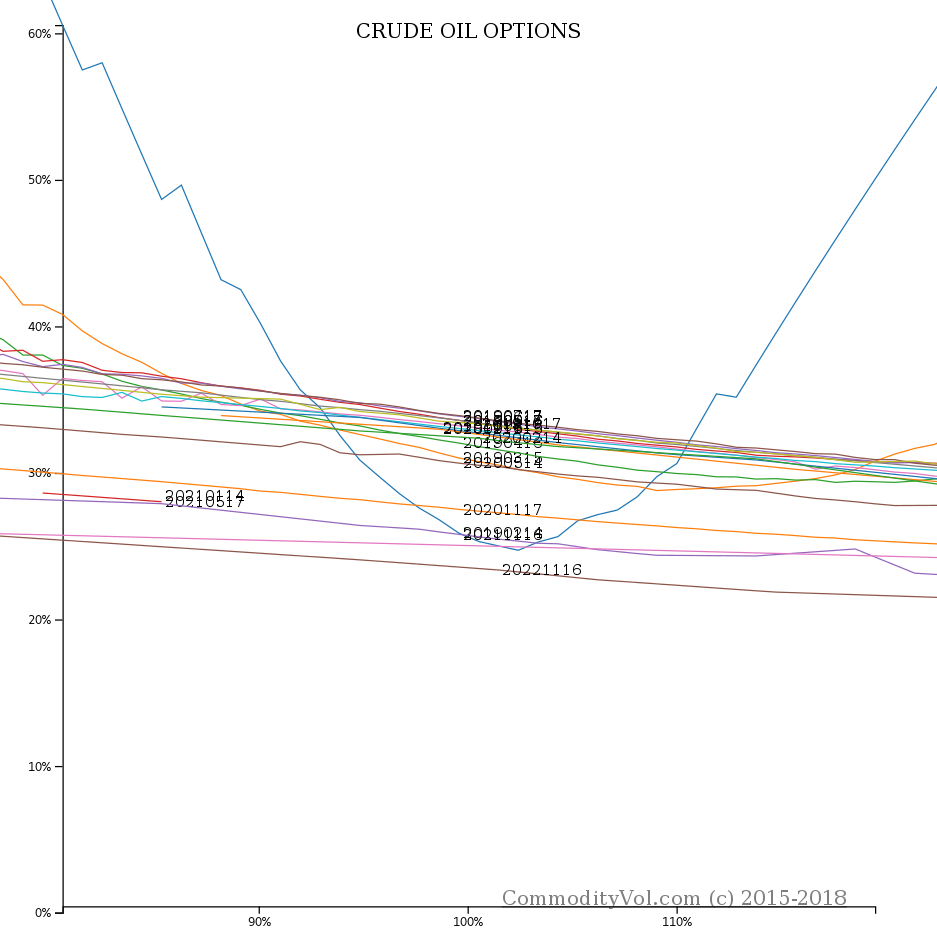### NYMEX LO Feb 8, 2019#### At the money vols and strikes (approx)

• LO G 2019 Vol= 0.2589 Strike= 52.5
• LO H 2019 Vol= 0.3077 Strike= 53
• LO J 2019 Vol= 0.3157 Strike= 53.5
• LO K 2019 Vol= 0.3298 Strike= 54
• LO M 2019 Vol= 0.3315 Strike= 54.5
• LO N 2019 Vol= 0.3311 Strike= 55
• LO Q 2019 Vol= 0.3244 Strike= 55
• LO U 2019 Vol= 0.3276 Strike= 55
• LO V 2019 Vol= 0.3279 Strike= 55
• LO X 2019 Vol= 0.3219 Strike= 55.5
• LO Z 2019 Vol= 0.3207 Strike= 55
• LO F 2020 Vol= 0.3191 Strike= 55
• LO G 2020 Vol= 0.3180 Strike= 55
• LO H 2020 Vol= 0.2848 Strike= 65
• LO J 2020 Vol= 0.2797 Strike= 65
• LO K 2020 Vol= 0.2992 Strike= 55
• LO M 2020 Vol= 0.2698 Strike= 65
• LO N 2020 Vol= 0.2658 Strike= 65
• LO Q 2020 Vol= 0.2617 Strike= 65
• LO U 2020 Vol= 0.2581 Strike= 65
• LO V 2020 Vol= 0.2546 Strike= 65
• LO X 2020 Vol= 0.2701 Strike= 54.5
• LO F 2021 Vol= 0.2845 Strike= 45
• LO K 2021 Vol= 0.2803 Strike= 45
• LO X 2021 Vol= 0.2548 Strike= 53.5
• LO X 2022 Vol= 0.2337 Strike= 53.5
• LO X 2023 Vol= 0.2657 Strike= 30.5
The information above is the product code, expiration month codethe exact last day of trading is in the brackets finally we have the expiry year. This is generally not the standard form for symbology. However, with some contracts we may have two or more expiries with the same contract month. The contracts may even share expiry year, so in order to disambiguate the contracts we show extra detail.The vol is next, and finally the actual strike.